# What is hydraulic diameter? How is it defined? What is it equal to for a circular pipe of diameter D?

Question-AnswerCategory: Fluid MechanicsWhat is hydraulic diameter? How is it defined? What is it equal to for a circular pipe of diameter D?

What is hydraulic diameter? How is it defined? What is it equal to for a circular pipe of diameter D?

Step 1 of 2
Hydraulic diameter is a parameter used to determine the Reynold’s number which determines the type of flow such as turbulent, laminar, and transition etc., in pipes having non-circular cross sections such as rectangular ducts, square pipes etc., used in air conditioners, heating ducts in buildings.
Hydraulic diameter is denoted as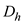. It is determined as the ratio of four times the area of the cross section to the perimeter of the cross section.
Write the formula for hydraulic diameter as,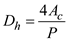Here, area of the cross section of the pipe is, and perimeter of the pipe is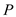..

Step 2 of 2

Determine the formula for hydraulic diameter a circular pipe using the relation,…… (1)
Write the formula for area of the cross section of the pipe.Here, diameter of the pipe is.
Write the perimeter of the cross section of the pipe.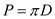Substitutefor, andforin equation (1).Therefore, hydraulic depth for a circular pipe is equal to its diameter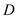.